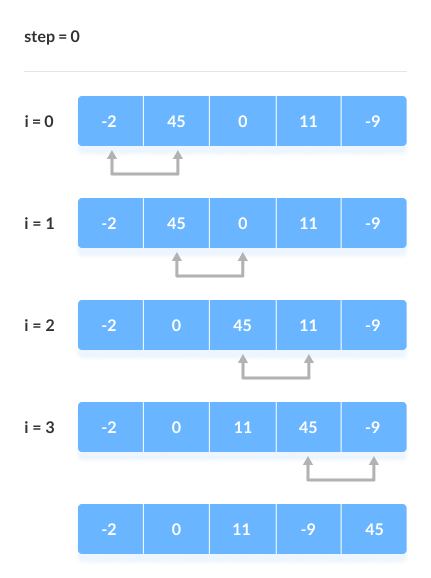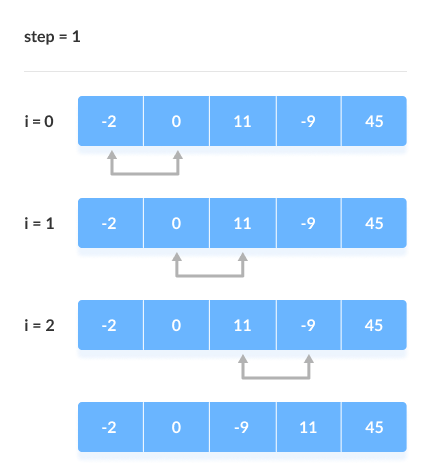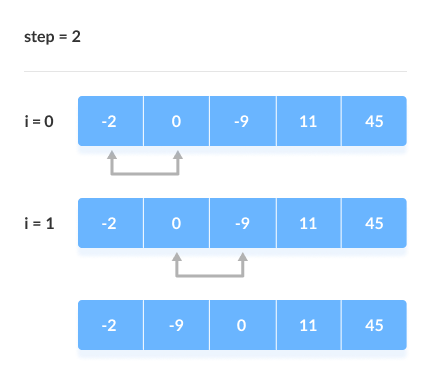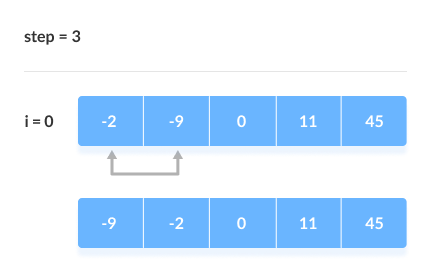Hits: 13

# Bubble Sort Algorithm

#### In this tutorial, you will learn how bubble sort works. Also, you will find working examples of bubble sort in Python.

Bubble sort is an algorithm that compares the adjacent elements and swaps their positions if they are not in the intended order. The order can be ascending or descending.

## How Bubble Sort Works?

1. Starting from the first index, compare the first and the second elements.If the first element is greater than the second element, they are swapped.Now, compare the second and the third elements. Swap them if they are not in order.

The above process goes on until the last element.Compare the adjacent elements
2. The same process goes on for the remaining iterations. After each iteration, the largest element among the unsorted elements is placed at the end.In each iteration, the comparison takes place up to the last unsorted element.

The array is sorted when all the unsorted elements are placed at their correct positions.Compare the adjacent elementsCompare the adjacent elementsCompare the adjacent elements

## Bubble Sort Algorithm

```bubbleSort(array)
for i <- 1 to indexOfLastUnsortedElement-1
if leftElement > rightElement
swap leftElement and rightElement
end bubbleSort
```

## Python Examples

``````/* Bubble sort in Python */

def bubbleSort(array):

/* run loops two times: one for walking throught the array
and the other for comparison */
for i in range(len(array)):
for j in range(0, len(array) - i - 1):

/* To sort in descending order, change > to < in this line. */
if array[j] > array[j + 1]:

/* swap if greater is at the rear position */
(array[j], array[j + 1]) = (array[j + 1], array[j])

data = [-2, 45, 0, 11, -9]
bubbleSort(data)
print('Sorted Array in Asc ending Order:')
print(data)``````

## Optimized Bubble Sort

In the above code, all possible comparisons are made even if the array is already sorted. It increases the execution time.

The code can be optimized by introducing an extra variable swapped. After each iteration, if there is no swapping taking place then, there is no need for performing further loops.

In such a case, variable swapped is set false. Thus, we can prevent further iterations.

Algorithm for optimized bubble sort is

```bubbleSort(array)
swapped <- false
for i <- 1 to indexOfLastUnsortedElement-1
if leftElement > rightElement
swap leftElement and rightElement
swapped <- true
end bubbleSort
```

### Optimized Bubble Sort Examples

``````/* Optimized bubble sort in python */

def bubbleSort(array):

/* Run loops two times: one for walking throught the array
and the other for comparison */
for i in range(len(array)):

/* swapped keeps track of swapping */
swapped = True
for j in range(0, len(array) - i - 1):

/* To sort in descending order, change > to < in this line. */
if array[j] > array[j + 1]:

/* Swap if greater is at the rear position */
(array[j], array[j + 1]) = (array[j + 1], array[j])
swapped = False

/* If there is not swapping in the last swap, then the array is already sorted. */
if swapped:
break

data = [-2, 45, 0, 11, -9]
bubbleSort(data)
print('Sorted Array in Ascending Order:')
print(data)
``````

## Complexity

Bubble Sort is one of the simplest sorting algorithms. Two loops are implemented in the algorithm.

Cycle Number of Comparisons
1st (n-1)
2nd (n-2)
3rd (n-3)
……. ……
last 1

Number of comparisons: (n – 1) + (n – 2) + (n – 3) +…..+ 1 = n(n – 1) / 2 nearly equals to n2

Complexity: O(n2)

Also, we can analyze the complexity by simply observing the number of loops. There are 2 loops so the complexity is `n*n = n2`

Time Complexities:

• Worst Case Complexity:`O(n2)`
If we want to sort in ascending order and the array is in descending order then, the worst case occurs.
• Best Case Complexity:`O(n)`
If the array is already sorted, then there is no need for sorting.
• Average Case Complexity:`O(n2)`
It occurs when the elements of the array are in jumbled order (neither ascending nor descending).

Space Complexity:
Space complexity is `O(1)` because an extra variable temp is used for swapping.

In the optimized algorithm, the variable swapped adds to the space complexity thus, making it `O(2)`.

## Bubble Sort Applications

Bubble sort is used in the following cases where

1. the complexity of the code does not matter.
2. a short code is preferred.

# Special 95% discount

## 2000+ Applied Machine Learning & Data Science Recipes

### Portfolio Projects for Aspiring Data Scientists: Tabular Text & Image Data Analytics as well as Time Series Forecasting in Python & R## Two Machine Learning Fields

There are two sides to machine learning:

• Practical Machine Learning:This is about querying databases, cleaning data, writing scripts to transform data and gluing algorithm and libraries together and writing custom code to squeeze reliable answers from data to satisfy difficult and ill defined questions. It’s the mess of reality.
• Theoretical Machine Learning: This is about math and abstraction and idealized scenarios and limits and beauty and informing what is possible. It is a whole lot neater and cleaner and removed from the mess of reality.

Data Science Resources: Data Science Recipes and Applied Machine Learning Recipes

Introduction to Applied Machine Learning & Data Science for Beginners, Business Analysts, Students, Researchers and Freelancers with Python & R Codes @ Western Australian Center for Applied Machine Learning & Data Science (WACAMLDS) !!!

Latest end-to-end Learn by Coding Recipes in Project-Based Learning:

Applied Statistics with R for Beginners and Business Professionals

Data Science and Machine Learning Projects in Python: Tabular Data Analytics

Data Science and Machine Learning Projects in R: Tabular Data Analytics

Python Machine Learning & Data Science Recipes: Learn by Coding

R Machine Learning & Data Science Recipes: Learn by Coding

Comparing Different Machine Learning Algorithms in Python for Classification (FREE)

`Disclaimer: The information and code presented within this recipe/tutorial is only for educational and coaching purposes for beginners and developers. Anyone can practice and apply the recipe/tutorial presented here, but the reader is taking full responsibility for his/her actions. The author (content curator) of this recipe (code / program) has made every effort to ensure the accuracy of the information was correct at time of publication. The author (content curator) does not assume and hereby disclaims any liability to any party for any loss, damage, or disruption caused by errors or omissions, whether such errors or omissions result from accident, negligence, or any other cause. The information presented here could also be found in public knowledge domains.  `